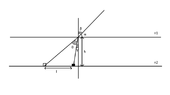# Geometrical optics: using Snell's law, find the depth of the pool

• tomceka

#### tomceka

Homework Statement
A person looks at the surface of water with an angle of 30 degrees and sees a coin 0.5 m further than it really is. What is the depth (h) of the pool.
The answer I should get is around 16 cm, but I get 0.289 m. What is wrong with my solution?
Relevant Equations
Snell's law; basic trigonometry
α=30°; l=0.5 m; n1=1; n2=1.33

α+β=90°, so β=90°-30°=60°.
Using Snell's law:
sinβ/sinγ = n2/n1
sinγ≈0.651
γ≈41°.

β=γ+θ (vertical angles)
θ=60°-41°=19°

tan(θ+β)=l/h
h=l/tan(θ+γ)
h=0.5/(tan(19+41))≈0.289 m

#### Attachments

•photos.png
2.6 KB · Views: 39
Last edited:
Welcome!
Why θ+β?

Welcome!
Why θ+β?
My bad, it should be θ+γ. Although the answer is still wrong. Is there something I missed?

Last edited:
The equation tan(θ+γ)=l/h is not correct.
The coin is resting at a point far from the vertical line.

Do you mind explaining what do you mean by that? Is there something wrong with my diagram?

Do you mind explaining what do you mean by that? Is there something wrong with my diagram?
The diagram is fine. It's your trig based on it that is not. Let x = distance from the coin to the vertical line. There is a right triangle that has angle γ and ##x## is opposite to it. Then the correct expression is, ##\tan(\theta+\gamma)=\dfrac{l+x}{h}##.

•Lnewqban
The diagram is fine. It's your trig based on it that is not. Let x = distance from the coin to the vertical line. There is a right triangle that has angle γ and ##x## is opposite to it. Then the correct expression is, ##\tan(\theta+\gamma)=\dfrac{l+x}{h}##.
Thank you.